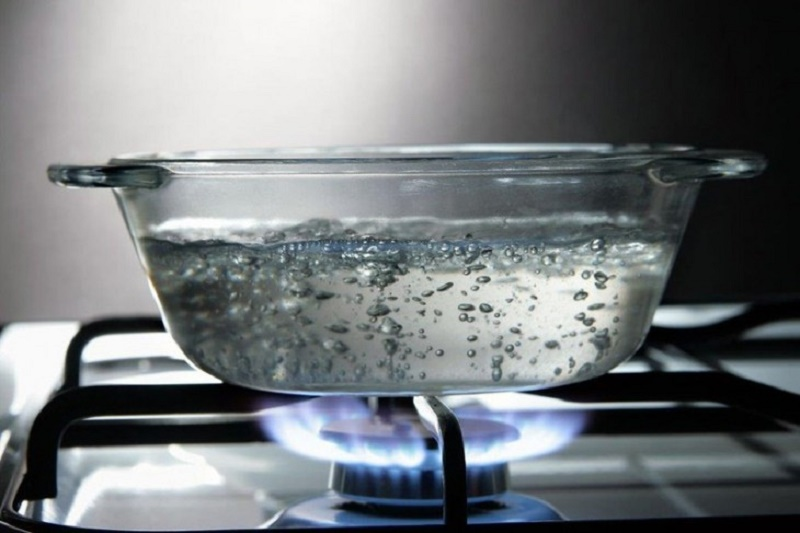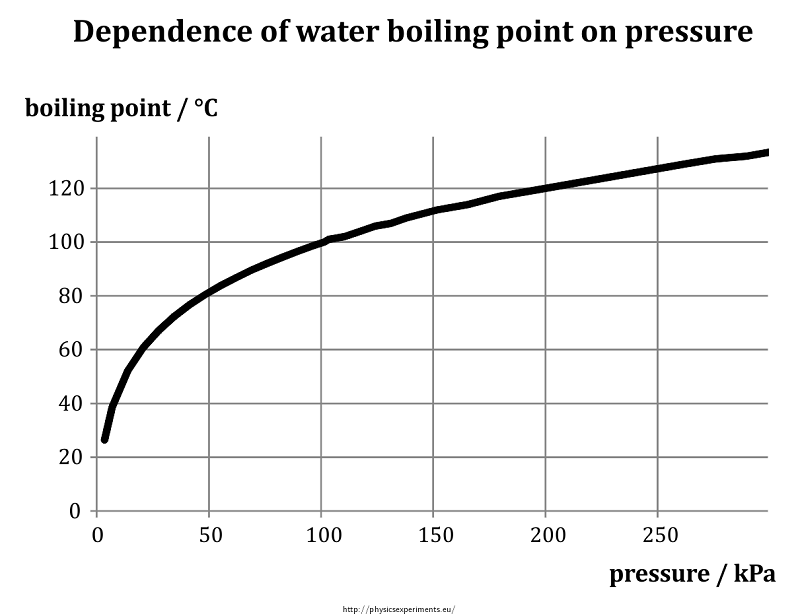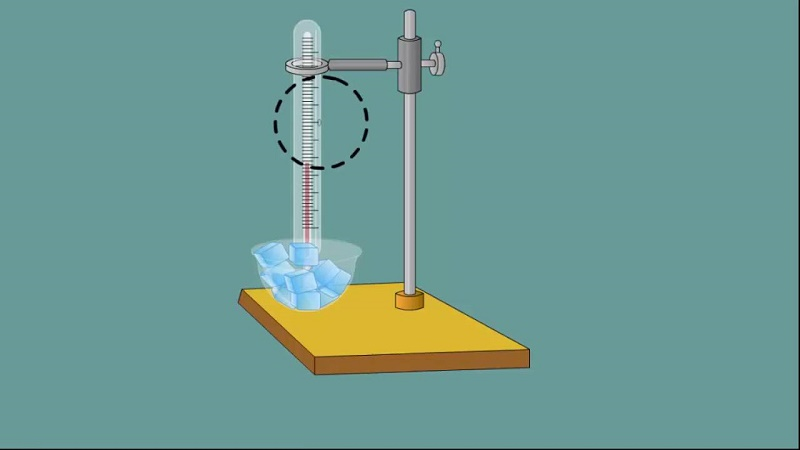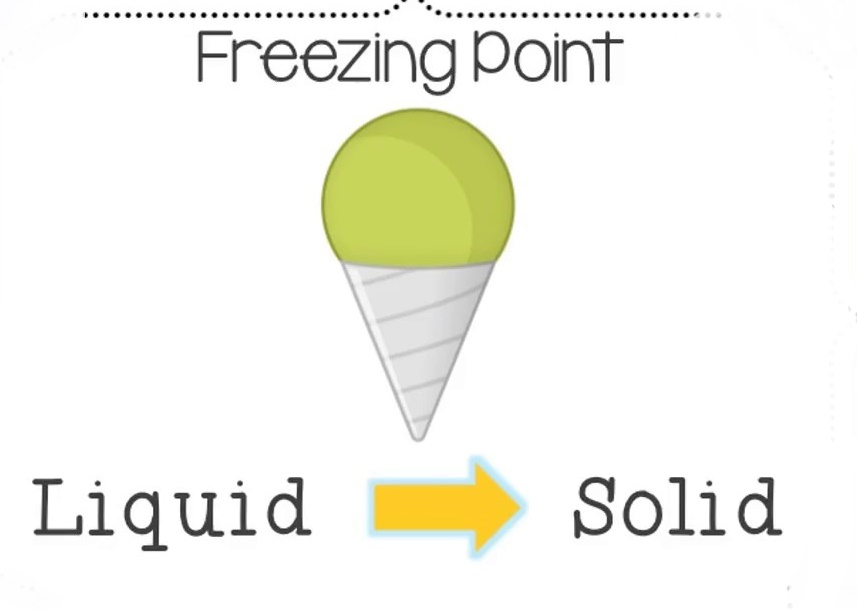# What is the boiling point and write down the formula to calculate

What is the boiling point? The boiling point is the temperature at which the vapor pressure of a liquid reaches that of the medium. In other words, the temperature at which a liquid leaves its state and enters the gaseous state (vapor).

This point is reached by injecting heat into the physical system of the liquid. Its particles agitate more rapidly and register a considerable increase in entropy (tendency to disorder the system). As the particles located near the surface break the surface tension and they escape, converted into vapor.

## The boiling pointDifferent substances have different boiling points since this depends on the molecular mass of the substance and the type of intermolecular forces that present: covalent bonds (polar or non-polar) or hydrogen bonds, among others.

The speed with which this point is reached also depends on factors such as pressure since the pressure is accelerated when the pressure increases. On the other hand, the boiling point represents a maximum stop, since the temperature can not increase beyond it since there would be no more liquid to heat.

### How is it calculated?

The formula for calculating the boiling point of substances is the Clausius-Clapeyron formula, which reads as follows:

T B = ([R ln (P 0 ) / ΔH vap ] + [1 / T 0 ]) -1

And where T B is the normal boiling point expressed in degrees Kelvin, R is the constant gas equivalent to 8,314 JK -1 .mol – 1, P 0 is the vapor pressure expressed in atmospheres, ΔH map is the enthalpy of vaporization expressed in J / mol, T 0 is the temperature in degrees Kelvin at which the vapor pressure is measured, and ln is the natural logarithm.#### Examples

Some boiling points registered and known under normal pressure conditions (1 atm) are the following:

• Water: 100 ° C
• Helium: -268.9 ° C
• Hydrogen: -252.8 ° C
• Calcium: 1484 ° C
• Beryllium: 2471 ° C
• Silicon: 3265 ° C
• Carbon: 3825 ° C
• Boron: 4000 ° C
• Molybdenum: 4639 ° C
• Osmium: 5012 ° C
• Tungsten: 5555 ° C

### Melting pointThe melting point is, similarly, the maximum temperature reached by a substance in the solid state before it enters the liquid state. It operates similarly to boiling: the system gains heat until the compact space of the solid structure is too small to contain the movement of the particles, which are held together but now with a unique fluidity and fluidity.

This point is also affected by the pressure of the system. This is what happens, for example, when melting ice.

### Freezing pointThe freezing point is the opposite of the melting point, that is, the temperature at which a liquid contracts, loses molecular movement and acquires a more rigid structure, resistant to deformation and memory of form, unique to the substances in the solid state. If fusion requires injecting heat energy into the system, freezing requires the withdrawal of heat energy (cooling).

Again, the pressure to which the substance is placed must be taken into account. This is what happens when you freeze water and get ice, for example.

#### The melting point and boiling water

The water is often used as a standard when measuring the melting points and boiling substances. And in general, at normal pressure, its boiling point is 100 ° C, and the melting point is 0 ° C (in the case of ice). This can vary greatly, however, in cases where the water has other substances dissolved in it, liquid or solid. In the case with sea water, rich in salts, which modifies its physical and chemical properties.

The impact of the pressure is also very noticeable. It is known that at 1 atm the boiling point of water is 100 ° C, but taking it to 0.06 atmospheres, we would be surprised to notice that boiling occurs at 0 ° C (instead of freezing).

You may also like What is Windows Code Injection and why it can be dangerous